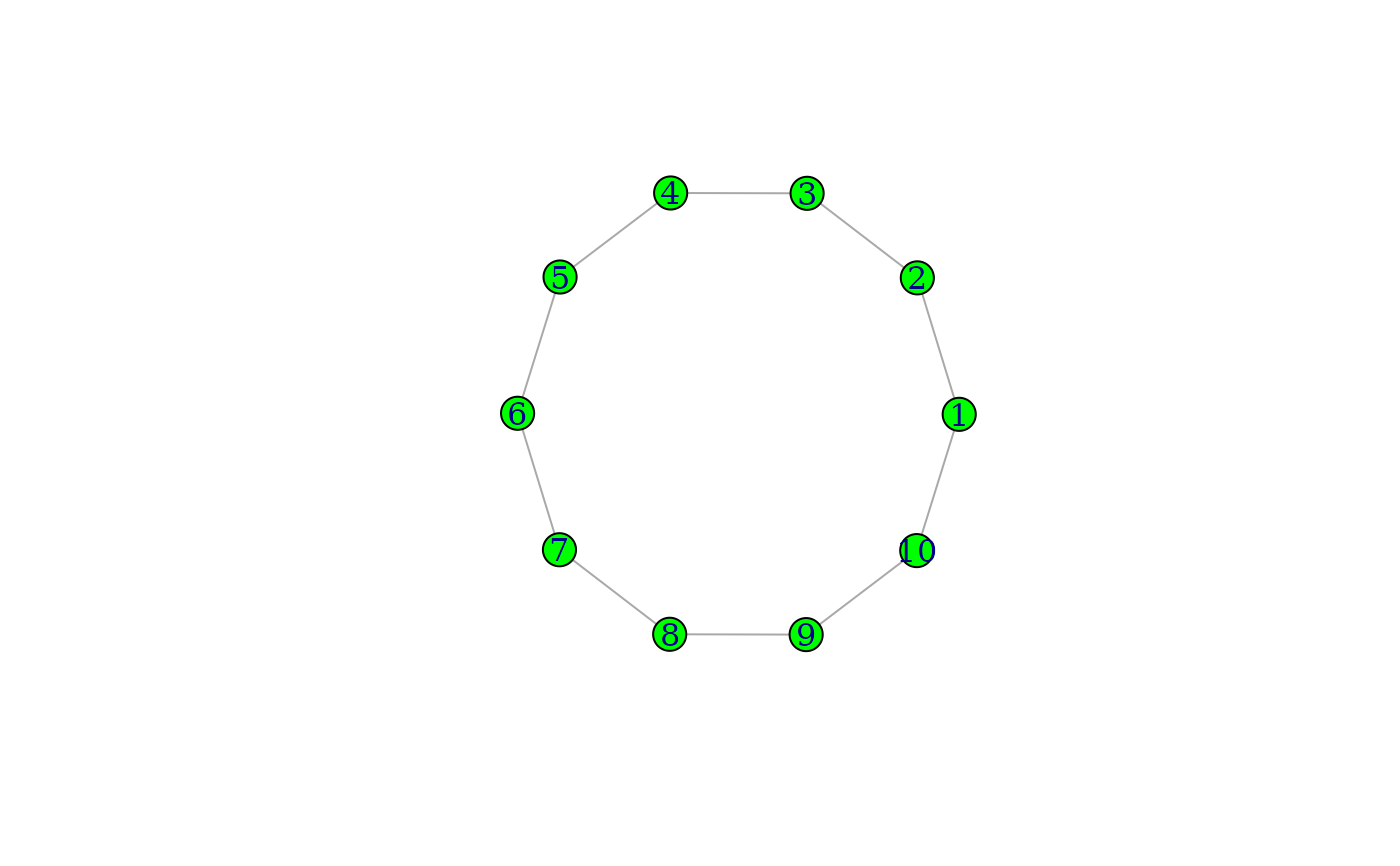plot.igraph() is able to plot graphs to any R device. It is the non-interactive companion of the tkplot() function.

## Usage

# S3 method for igraph
plot(
x,
axes = FALSE,
xlim = c(-1, 1),
ylim = c(-1, 1),
mark.groups = list(),
mark.shape = 1/2,
mark.col = rainbow(length(mark.groups), alpha = 0.3),
mark.border = rainbow(length(mark.groups), alpha = 1),
mark.expand = 15,
loop.size = 1,
...
)

## Arguments

x

The graph to plot.

axes

Logical, whether to plot axes, defaults to FALSE.

Logical scalar, whether to add the plot to the current device, or delete the device's current contents first.

xlim

The limits for the horizontal axis, it is unlikely that you want to modify this.

ylim

The limits for the vertical axis, it is unlikely that you want to modify this.

mark.groups

A list of vertex id vectors. It is interpreted as a set of vertex groups. Each vertex group is highlighted, by plotting a colored smoothed polygon around and “under” it. See the arguments below to control the look of the polygons.

mark.shape

A numeric scalar or vector. Controls the smoothness of the vertex group marking polygons. This is basically the ‘shape’ parameter of the graphics::xspline() function, its possible values are between -1 and 1. If it is a vector, then a different value is used for the different vertex groups.

mark.col

A scalar or vector giving the colors of marking the polygons, in any format accepted by graphics::xspline(); e.g. numeric color ids, symbolic color names, or colors in RGB.

mark.border

A scalar or vector giving the colors of the borders of the vertex group marking polygons. If it is NA, then no border is drawn.

mark.expand

A numeric scalar or vector, the size of the border around the marked vertex groups. It is in the same units as the vertex sizes. If a vector is given, then different values are used for the different vertex groups.

loop.size

A numeric scalar that allows the user to scale the loop edges of the network. The default loop size is 1. Larger values will produce larger loops.

...

Additional plotting parameters. See igraph.plotting for the complete list.

## Value

Returns NULL, invisibly.

## Details

One convenient way to plot graphs is to plot with tkplot() first, handtune the placement of the vertices, query the coordinates by the tk_coords() function and use them with plot() to plot the graph to any R device.

layout() for different layouts, igraph.plotting for the detailed description of the plotting parameters and tkplot() and rglplot() for other graph plotting functions.

Other plot: rglplot()

## Author

Gabor Csardi csardi.gabor@gmail.com

## Examples


g <- make_ring(10)
plot(g, layout = layout_with_kk, vertex.color = "green")